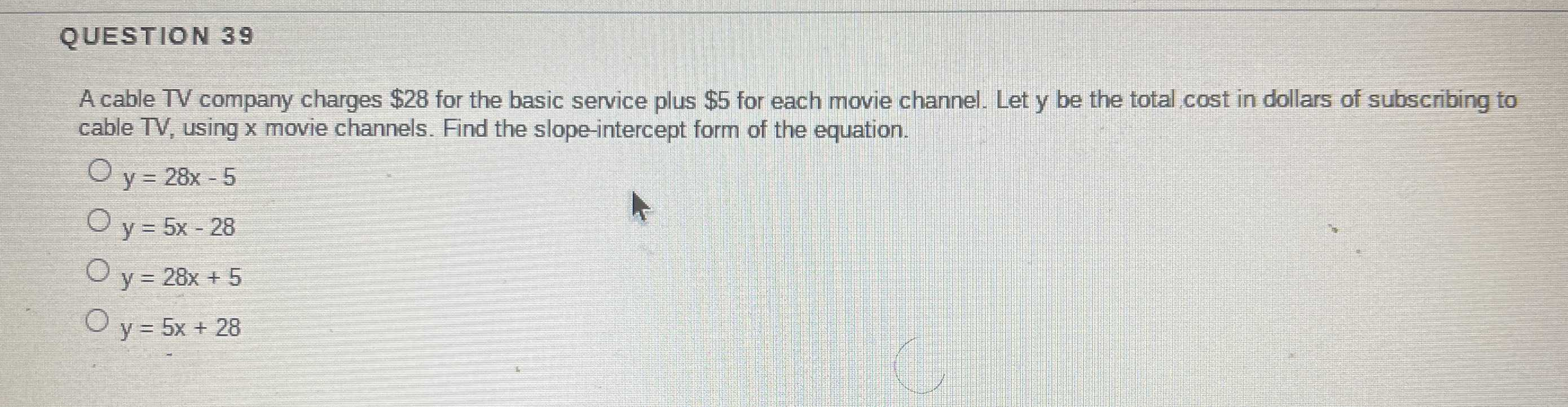### ¿Todavía tienes preguntas de matemáticas?

Pregunte a nuestros tutores expertos
Algebra
PreguntaA cable TV company charges $$\ 28$$ for the basic service plus $$\ 5$$ for each movie channel. Let $$y$$ be the total cost in dollars of subscribing to cable TV, using $$x$$ movie channels. Find the slope-intercept form of the equation.

$$y = 28 x - 5$$

$$y = 5 x - 28$$

$$y = 28 x + 5$$

$$y = 5 x + 28$$